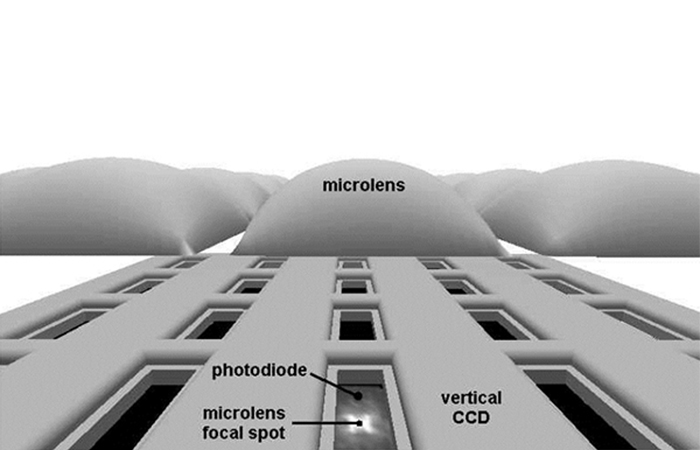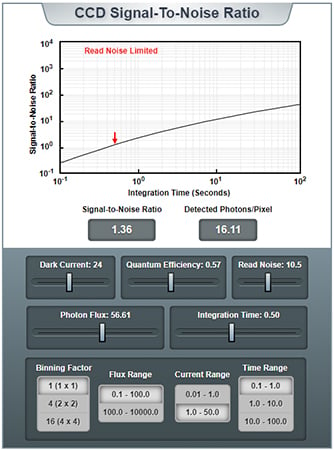Technical Article

# Quantum Efficiency and Signal-to-Noise Ratio in CCD Image Sensors

May 25, 2020 by Robert Keim

## In this article, we’ll draw upon our knowledge of noise sources and combine it with some new information to create a comprehensive model for CCD noise performance.

This article is Part 13 of the AAC series on CCD (charge-coupled device) image sensors. Before we dive into quantum efficiency and signal-to-noise ratio of CCD image sensors, you may find it helpful to catch up on this ongoing conversation:

In order to determine the overall signal-to-noise ratio (SNR) of a CCD imaging system, we need to know the magnitude of the signal generated in response to incident light and the total noise. In the previous two articles, we explored dark noise, photon noise, read noise, and reset noise.

We’re going to assume that the CCD’s output signal is processed using correlated double sampling (more on this in our article on sampling, amplifying, and digitizing CCD output signals) and consequently we can ignore reset noise.

### Calculating the CCD Signal Level

Three factors determine the amount of signal generated by a CCD. Two of the three are the intensity of the incident illumination and the duration of the incident illumination.

The official name for illumination intensity is photon flux (denoted by P), and it is quantified as the number of photons that arrive at a given pixel location per second. If we multiply this number by the integration time (denoted by t), we know the total number of photons that a pixel received during the image-formation period.

#### Quantum Efficiency

If a CCD pixel could consistently produce one free electron for every incident photon, we could calculate the signal level using only the quantities mentioned above.

However, CCDs, like human beings, are imperfect. For various reasons, not every photon produces a usable electron. Some are absorbed, scattered, or reflected by polysilicon electrodes, some might produce electrons that diffuse directly into an anti-blooming CCD structure, and others may strike non-light-sensitive portions of the pixel.

This is why we need a third factor: quantum efficiency (QE, or QE in equations). This value specifies the CCD’s overall sensitivity to light, and it allows us to calculate how much usable electric charge is generated in relation to the total number of incident photons.##### Interline-transfer CCDs without microlenses would have low QE, because a large portion of the pixel area is not photoactive. Microlenses direct photons to the photoactive region, but they also create some reflection and absorption. Graphic used courtesy of ON Semiconductor

Quantum efficiency of 100% would indicate that every photon is converted into a usable electron. Back-illuminated CCDs can achieve QE greater than 90% at certain wavelengths, but a typical front-illuminated CCD will have an average visible-spectrum QE that is significantly lower.

If a pixel with QE = 30% receives 1000 photons during an integration period, the light-generated charge packet in that pixel will consist of 300 electrons, and this becomes the signal term (S) in our SNR calculation. Thus, signal equals photon flux multiplied by integration time multiplied by quantum efficiency:

$S=PtQ_E$

### The CCD SNR Formula

The most important noise sources in CCD operation are photon noise, dark noise, and read noise.

• Photon noise is calculated as the square root of the photon signal level. Thus, $$N_P = \sqrt(PtQE)$$.
• Dark noise is calculated as the square root of dark current multiplied by the integration time: $$N_D = \sqrt(I_Dt)$$.
• Read noise (NR) isn’t calculated from another readily available quantity. You have to find a read noise spec.

$SNR=\frac{PtQ_E}{N_P+N_D+N_R}$

$SNR=\frac{PtQ_E}{\sqrt{PtQ_E+{I_Dt}+N_R^2}}$

One thing to keep in mind is that QE varies significantly as a function of wavelength. Thus, to increase the accuracy of the calculated signal level (and, consequently, of the calculated photon noise), you would need to account for the CCD’s sensitivity to the optical wavelengths that are present in a given application or operational environment.

### A Handy Tool for CCD SNR

Nikon created an online calculator that makes quick work of SNR analysis and also produces an informative plot of SNR vs. integration time.##### CCD SNR calculator. Image used courtesy of Nikon

If you play around with the sliders, you’ll see that longer integration time consistently produces higher SNR. Also note that the tool allows you to easily determine how binning will influence the overall SNR.

If you experiment with the tool, you’ll notice that sometimes it indicates “Read Noise Limited” and other times it indicates “Photon Noise Limited.” Let’s briefly discuss this issue before we conclude this article.

### Read-Noise Limited vs. Photon-Noise Limited

If a CCD’s total noise is dominated by read noise, we say that it is read-noise limited. If the total noise is dominated by photon-noise, it’s photon-noise limited. More incident light leads to more photon noise; thus, photon noise tends to dominate when the system has longer integration time and higher photon flux.

#### Dark-Noise Limited?

It seems possible that a CCD could be dark-noise limited since the amount of dark noise can be relatively large at higher temperatures. Nevertheless, we don’t really have a “dark-noise limited” category.

First, dark noise tends to be small, especially with the integration times used in typical photography and videography. The KAI-2020, for example, has about 20 electrons RMS of read noise. At 40°C and with an integration time of 100 ms (which is a fairly long exposure for everyday image capture), the dark noise would be 2 electrons RMS (typical) and 5.9 electrons RMS (maximum).

Furthermore, dark noise is never a limiting factor in a purely experimental context, because we can always reduce dark noise to negligible levels by cooling the sensor.

### Conclusion

If we know photon flux, dark current, and read noise, we can estimate the SNR of a CCD’s output signal using a fairly straightforward formula.

In the next article, we’ll explore dynamic range, which is a related concept that also plays an important role in the image quality produced by a CCD camera system.